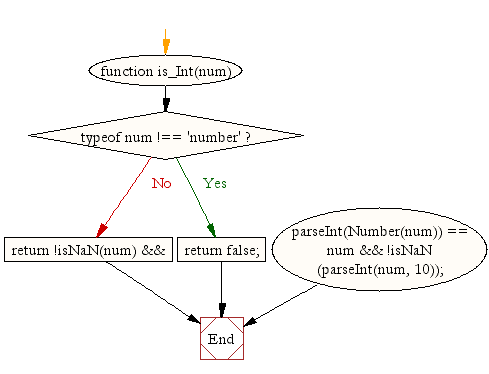# JavaScript: Check whether a value is an integer or not

## JavaScript Math: Exercise-15 with Solution

Write a JavaScript function to check whether a value is an integer or not.

Test Data:
console.log(is_Int(23));
console.log(is_Int(4e2));
console.log(is_Int(NaN));
console.log(is_Int(23.75));
console.log(is_Int(-23));
Output :
true
true
false
false
true

Pictorial Presentation:Sample Solution:-

HTML Code:

``````<!DOCTYPE html>
<html>
<meta charset="utf-8">
<title>Check whether a value is an integer or not</title>
<body>
</body>
</html>
```
```

JavaScript Code:

``````function is_Int(num) {

if (typeof num !== 'number')
return false;

return !isNaN(num) &&
parseInt(Number(num)) == num &&
!isNaN(parseInt(num, 10));
}
console.log(is_Int(23));
console.log(is_Int(4e2));
console.log(is_Int(NaN));
console.log(is_Int(23.75));
console.log(is_Int(-23));
```
```

Sample Output:

```true
true
false
false
true
```

Flowchart:Live Demo:

See the Pen javascript-math-exercise-15 by w3resource (@w3resource) on CodePen.

Improve this sample solution and post your code through Disqus

What is the difficulty level of this exercise?

Test your Programming skills with w3resource's quiz.

﻿